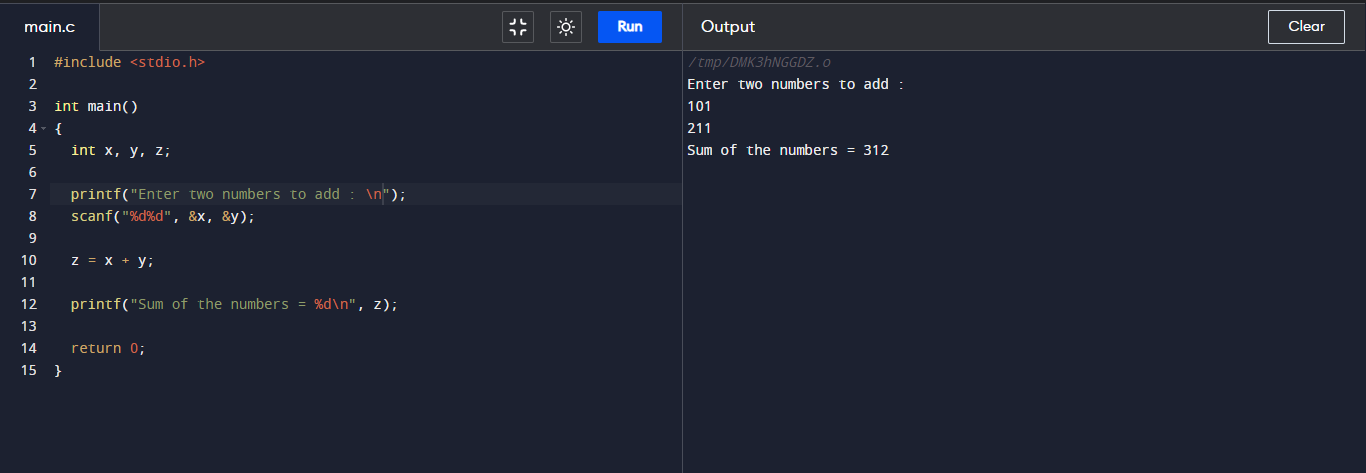C Program to add Two numbers

How can we add two numbers in C? We can add numbers using arithmetic operator "+" and print their sum using 'printf' function.

Addition of two numbers in C :

#include <stdio.h> int main()
{ int x, y, z; printf("Enter two numbers to add : \n"); scanf("%d%d", &x, &y); z = x + y; printf("Sum of the numbers = %d\n", z); return 0; }

Output :